# SBI Clerk Prelims Reasoning Ability Questions 2021 (Day-77)

Dear Aspirants, Our IBPS Guide team is providing new series of Reasoning Questions for SBI Clerk Prelims 2021 so the aspirants can practice it on a daily basis. These questions are framed by our skilled experts after understanding your needs thoroughly. Aspirants can practice these new series questions daily to familiarize with the exact exam pattern and make your preparation effective.

Start Quiz

Unknown based linear

Direction (1-5): Study the following information carefully and answer the below questions.

A certain number of persons are sitting in a row facing north. Three persons are sitting between M and G. L sits second to the right of R. T sits third to the left of M. O sits exactly between G and R. X sits at the end of the row. The number of seats between G and M is the same as between G and O. Not more than two persons are sitting between X and T. The number of persons sitting to the left of T is one more than the right of R.

1) What is the position of T with respect to O?

A.Ninth to the right

B.Eighth to the left

C.Tenth to the left

D.Eleventh to the left

E.Fifth to the right

2) How many persons are sitting in the row?

A.22

B.21

C.17

D.20

E.19

3) Four of the following five are similar in such a way that they form a group, who among the following doesn’t belong to the group?

A.M

B.G

C.the one who sits third to the right of X

D.O

E.the one who sits second to the right of R

4) Who among the following person sits sixth to the left of G?

A.the one who sits third to the right of X

B.None of these

C.M

D.O

E.the one who sits second to the right of R

5) Which of the following statement is/are false?

I). Only five seats are between M and X

II). T sits exactly between M and X

III). More than six persons are sitting to the right of O.

A.Both I and III

B.Only III

C.Both I and II

D.Only II

E.None of the statement is false

Direction sense

Direction (6-8): Study the following information carefully and answer the below questions

Point P is 2m north of Point Q. Point Z is 9m west of Point Q. Point L is 15m east of Point M which is 20m south of Point Z. Point R is 3m south of Point L. Point T is west of point R and South of Point P.

6) How far and what is the direction of Q with respect to T?

A.21m towards the south

B.20m towards the south

C.23m towards the north

D.22m towards the north

E.None of the above

7) What is the shortest distance between Point L and Point Z?

A.21m

B.24m

C.20m

D.23m

E.25m

8) What is the shortest distance between T and R?

A.6m

B.15m

C.7m

D.9m

E.None of the above

Inequality

Direction (9-10): In these questions, the relationship between different elements is shown in the statements. These statements are followed by two conclusions. Find the conclusion which logically follows.

(a) If only conclusion I follow.

(b) If only conclusion II follows.

(c) If either conclusion I or II follows.

(d) If neither conclusion I nor II follows.

(e) If both conclusion I and II follow.

9) Statements:

P ≥ N = G > T < K > L

Conclusion:

I). G < K

II). P > T

10) Statements:

Q > K > L ≤ C = H > I

Conclusion:

I). K > I

II). H > L

Directions (1-5) :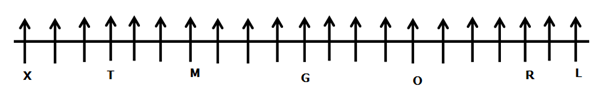Three persons are sitting between M and G.

T sits third to the left of M.

From the above condition, there are two possibilities.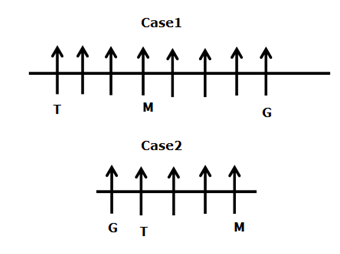The number of seats between G and M is the same as between G and O.

O sits exactly between G and R.

L sits second to the right of R.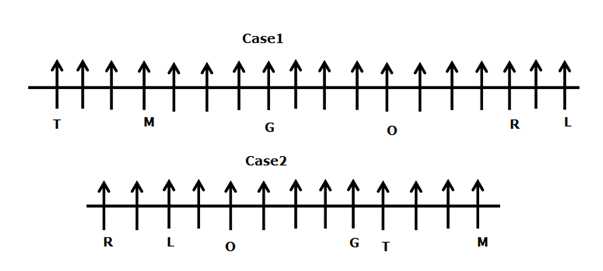The number of persons sitting to the left of T is one more than the right of R.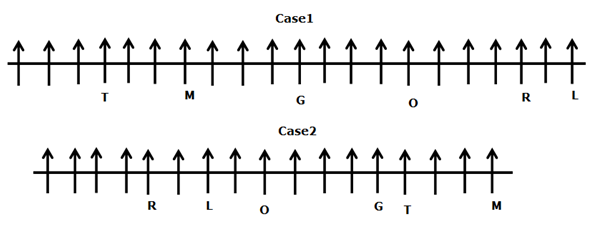X sits at the end of the row.

Not more than two persons are sitting between X and T.

From the above condition, case2 gets eliminated. case1 shows the final arrangement.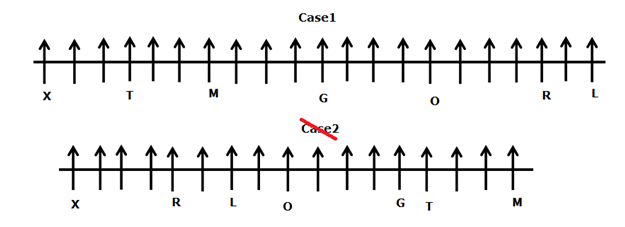Directions (6-8) :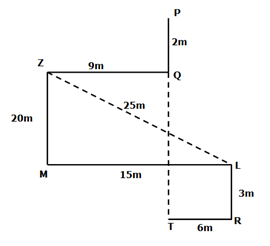202+152=ZL2

√(202+152)=√625=25m